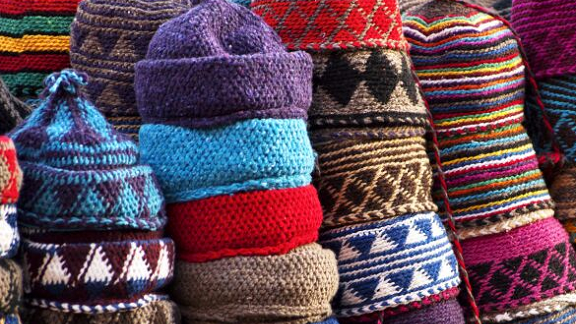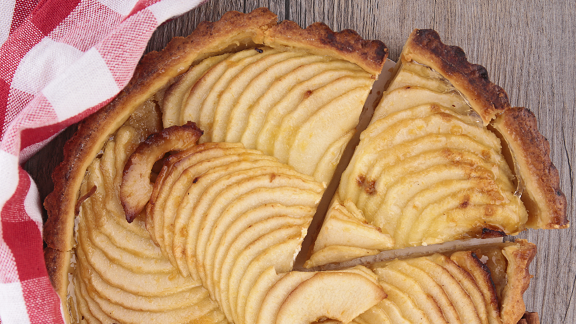# Special numbers

In some senses, all numbers are special, though some are perhaps given more attention than others... In this pathway, we invite you to take a closer look at some familiar categories of numbers and operations. We hope you'll discover that there's a lot more to averages, fractions and square numbers than you might initially imagine!### What's Possible?

What is special about numbers that can be written as the difference of two squares?### Equal Averages

Can you find sets of numbers whose mode, mean, median and range are all the same?### Averages

In these activities, you can be playful with averages, looking for patterns in the way the mean, median and mode change when you vary the numbers in a set.### Fractions

The world of fractions is much more interesting than you might imagine! We've collected together activities where you can explore properties of fractions, and make some unexpected discoveries about the world of rational numbers.### Square Numbers

If you can add, subtract and multiply, you'll be able to investigate square numbers and discover some famous results.